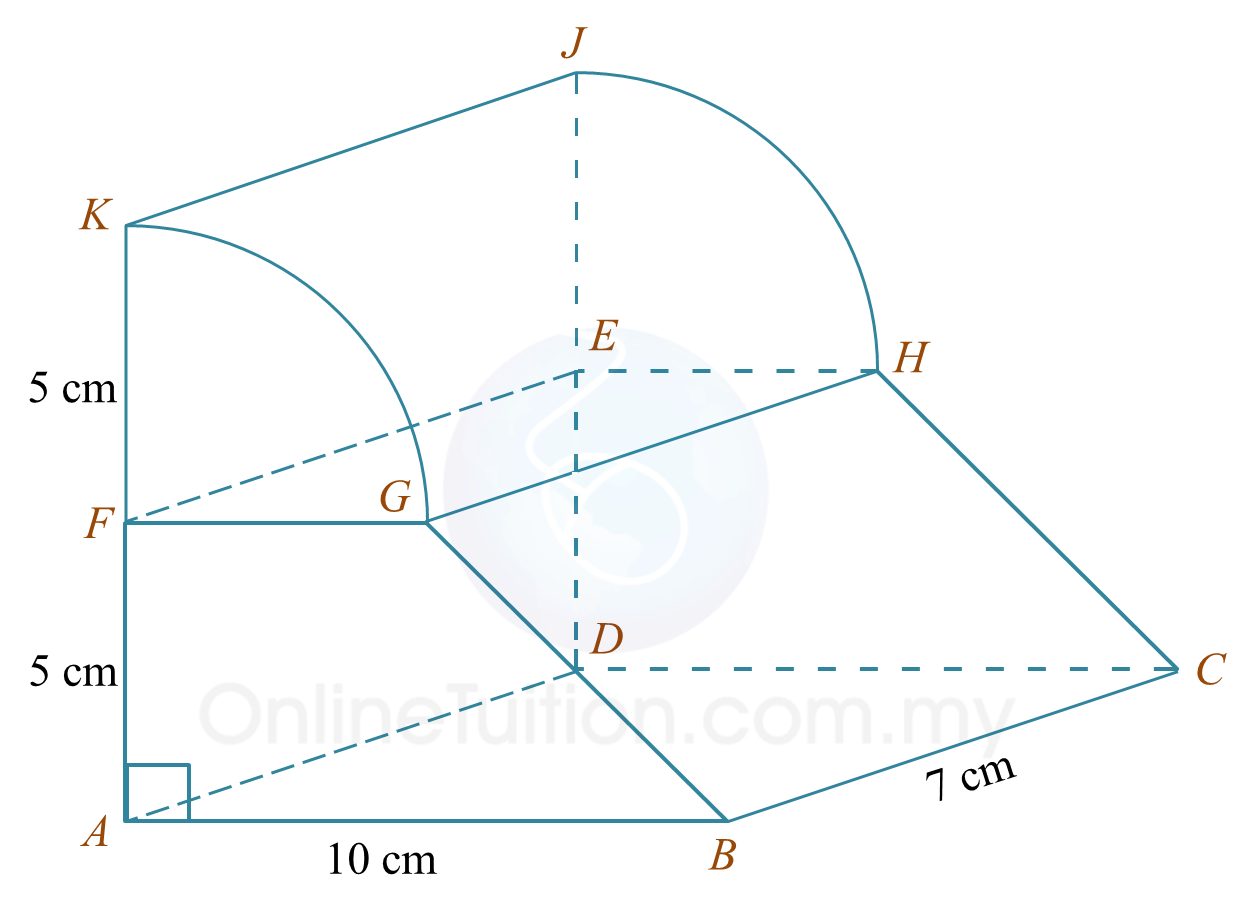# 6.5.4 Volume of Three Dimensional Shapes, PT3 Practice

Question 11:
Diagram shows a composite formed by joining a quarter cylinder and a right prism at the rectangular plane EFGH. The trapezium ABGF and the quarter circle FGK are the uniform cross sections of the solid.Using   $\pi =\frac{22}{7}$ , calculate the volume, in  cm3, of the composite solid.

Solution:

Question 12:
Diagram shows a cylindrical tank in a residential area which have 125 houses. Each house received equal volume of water.The diameter of the cylindrical tank is 4 m. It is given that each house has a cuboid tank with a base area of 0.8 m2.
Using  $\pi =\frac{22}{7}$ , calculate the height of the water level, in m, of each tank in the house.

Solution: